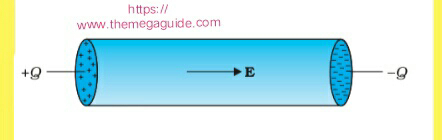Views

# Introduction :

Electric Current  is defined as the amount of electric charge flowing through any cross section of a conductor per unit time. Or It  is defined as the rate of flow of electric charge through cross section of a conductor.Electric Current

Let charge Q crosses through a cross section of a conductor in time "t", then

Current,

I = Q/t

## Current  in conductors :

We know that every matter is made up of atoms and molecules. In a solid conductor atoms and molecules are closely packed. The valence electrons of an atom are not attached firmly to its nucleus. These valence electrons are free to move through out the volume of the solid and hence known as free electrons.

Also, In a conductor free electrons move randomly that's in a Zig zag manner with a thermal speed of the order of 10 to 10 at room temperature.

In any portion of the conductor, the flow of electrons is so oriented that the number of electrons crossing through a given cross section of the conductor in any direction is equal to the number of electrons crossing through that Cross section of the conductor in opposite direction. In other words,  the flow of net electrons or charge through the given cross section of the conductor is zero. Hence,  Current in the conductor is zero.

### Now, let us apply electric field across the ends of a solid conductor in the form of cylinder as follows :

Connect a positively charged circular disc of a dielectric and of the same radius as that of the solid cylinder to one end of the cylinder. In the similar manner, connect negatively charged circular disc of a dielectric to the other end of the solid cylinder. The electric field is set up across the ends of the solid conductor from positive end to the negative end.

The free electron in the conductor experiences a force F=-eE  in the direction opposite to the direction of the electric field . Thus, free electrons in the solid conductors are accelerated in the direction opposite to the direction of the electric field. As the free electrons move from negative to positive disc, charges on the discs are neutralize.

Therefore, there is current in the conductor due to the movement of electrons from negative disc to positive disc. When the charges on the discs gets neutralize, the net flow of electrons from negative to positive discs stops and hence there is no current in the conductor. When both the discs get neutralize, there is no electric field across the conductor and no current in the conductor.

The  Current in a conductor exists only for the duration, the electric field exists across the conductor. In this particular situation, electric current exists only for short duration (as long as electric field exists). Such currents are known as transient currents.

However, if a steady electric field is applied across the solid conductor, then there will be steady current in the conductor. A steady electric field can be applied across the solid conductor by connecting a cell or a battery across the ends of the conductor . Thus, a cell or a battery connected across the ends of a solid conductor provides a steady current in the conductor.

#### Conclusion:

In conclusion,  Current flows in the conductor due to the presence of free electrons. The article has discussed about the flow of current in the conductor. It also tried to explain you this concept with little experiment